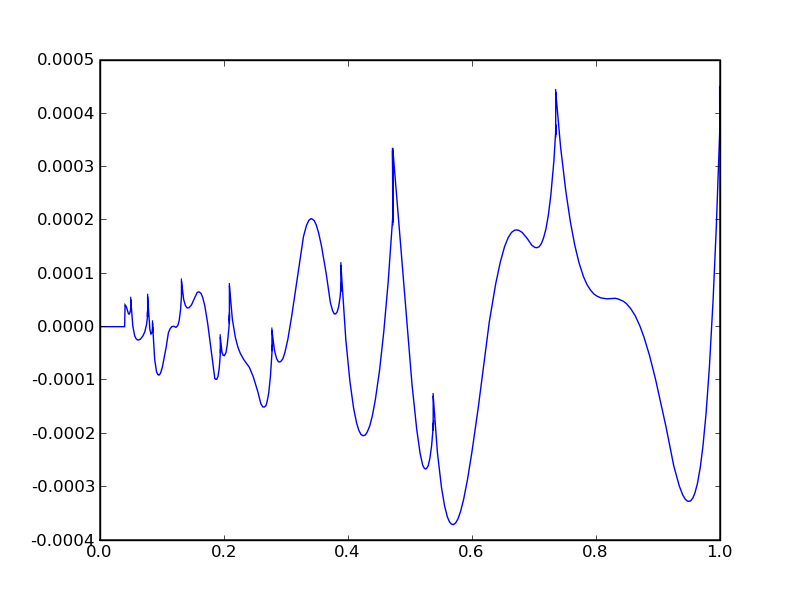Optimizing conversion between sRGB and linear

sRGB is a popular gamma-corrected color space.

These two functions follow from the sRGB definition:

def s2lin(x):
a = 0.055
return where(x <= 0.04045,
x * (1.0 / 12.92),
pow((x + a) * (1.0 / (1 + a)), 2.4))

def lin2s(x):
a = 0.055
return where(x <= 0.0031308,
x * 12.92,
(1 + a) * pow(x, 1 / 2.4) - a)

Implementation of pow is obviously important for performance. Using a Chebyshev approximation):

__c_exp2__ = Chebyshev(0, 1, 4, lambda x: math.pow(2, x))
__c_log2__ = Chebyshev(0.5, 1, 4, lambda x: math.log(x) / math.log(2))

def exp2(x):
xi = floor(x)
xf = x - xi
return ldexp(__c_exp2__.eval(xf), xi)

def log2(x):
(xf, xi) = frexp(x)
return xi + __c_log2__.eval(xf)

def pow(a, b):
return exp2(b * log2(a))

The SSE2 implementation runs in under 1ns per conversion on a modern Intel CPU.

The degree of the Chebyshev approximation needs to be as low as possible for performance. Here I needed to be accurate to within 10 bits, and found that degree 4 gives an error below 0.0005 for the crucial round-trip function:

lin2s(s2lin(x))Some ideas that did not work:

• Using Chebyshev approximations for the s2lin and lin2s functions. Only produced acceptable results with a degree of 20 or more, hence was quite slow.
• Approximations to x2.4 and x(1)/(2.4) using a sequence of square roots, (much like Optimizing pow()). Again, getting a result below the error bound took too long.